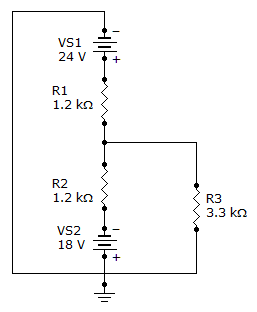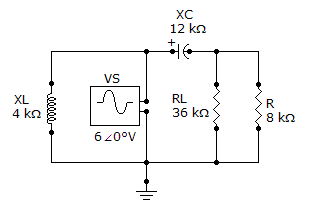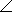# Online Electrical Engineering Test - Electrical Engineering Test 9Loading Test...

Instruction:

• This is a FREE online test. DO NOT pay money to anyone to attend this test.
• Total number of questions : 20.
• Time alloted : 30 minutes.
• Each question carry 1 mark, no negative marks.
• DO NOT refresh the page.
• All the best :-).

1.

The number 4.38 × 10–3 expressed as a number having a power of 10–6 is

A.
 4,380 × 10–6B.
 438 × 10–6C.
 43,800 × 10–6D.
 438,000 × 10–62.

The quantity 43 × 10-3 is the same as

A.
 0.043B.
 0.43C.
 430D.
 43,0003.

A certain appliance uses 350 W. If it is allowed to run continuously for 24 days, how many kilowatt-hours of energy does it consume?

A.
 20.16 kWhB.
 201.6 kWhC.
 2.01 kWhD.
 8.4 kWh4.

The following resistors (one each) are connected in a series circuit: 470, 680, 1 k, and 1.2 k. The voltage source is 20 V. Current through the 680resistor is approximately

A.
 60 mAB.
 30 mAC.
 6 mAD.
 300 mA5.

The sum of all the voltage drops around a single closed loop in a circuit is zero.

A.
 TrueB.
 False6.

Find the total current through R3 in the given circuit.A.
 7.3 mAB.
 5.5 mAC.
 12.8 mAD.
 1.8 mA7.

A phasor represents

A.
 the magnitude and a quantity directionB.
 the width of a quantityC.
 the phase angleD.
 the magnitude of a quantity8.

Of the following capacitors, which one has the highest dielectric constant?

A.
 airB.
 micaC.
 glassD.
 paper9.

Faraday's law states that relative motion between a magnetic field and a coil induces a voltage across the coil.

A.
 TrueB.
 False10.

Inductance is indirectly proportional to the square of the number of turns, the permeability, and the cross-sectional area of the core.

A.
 TrueB.
 FalseExplanation:

Some factors that affect the inductance of a coil include:

1. The inductance is proportional to the square of the number of turns;
2. The inductance increases directly as the permeability of the core material increases;
3. The inductance increases directly as the cross-sectional area increases; and
4. The inductance decreases as its length increases.

11.

If 25 W of power are applied to the primary of an ideal transformer with a turns ratio of 10, the power delivered to the secondary load is

A.
 25 WB.
 0 WC.
 250 WD.
 2.5 W12.

A transformer changes ac to dc.

A.
 TrueB.
 False13.

A filter passes all frequencies.

A.
 TrueB.
 False14.

To tune a parallel resonant circuit to a higher frequency, the capacitance should be

A.
 increasedB.
 decreasedC.
 left aloneD.
 replaced with inductance15.

A series resonant circuit is commonly called a tank circuit.

A.
 TrueB.
 False16.

Referring to the given circuit, find ZTH if R is 15 kand RL is 38 k.A.
 89.82–51.3° kB.
 19.2–38.3° kC.
 9.38–51.3° kD.
 180–38.3° k17.

In an ac circuit, power to the load peaks at the frequency at which the load impedance is the complex conjugate of the output impedance.

A.
 TrueB.
 False18.

The superposition theorem is useful for the analysis of single-source circuits.

A.
 TrueB.
 False19.

Like Thevenin's theorem, Norton's theorem provides a method of reducing a more complex circuit to a simpler, more manageable form for analysis.

A.
 TrueB.
 False20.

A balanced load is one in which all the impedances are equal.

A.
 TrueB.
 False